Courses

# Sample Test: Chemistry

## 20 Questions MCQ Test NEET Mock Test Series | Sample Test: Chemistry

Description
This mock test of Sample Test: Chemistry for NEET helps you for every NEET entrance exam. This contains 20 Multiple Choice Questions for NEET Sample Test: Chemistry (mcq) to study with solutions a complete question bank. The solved questions answers in this Sample Test: Chemistry quiz give you a good mix of easy questions and tough questions. NEET students definitely take this Sample Test: Chemistry exercise for a better result in the exam. You can find other Sample Test: Chemistry extra questions, long questions & short questions for NEET on EduRev as well by searching above.
QUESTION: 1

### The ratio of velocity of electron in 2nd to that in 4th excited state of Be3+ will be

Solution: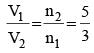QUESTION: 2

### An example of tranquilizer is

Solution:
• Meprobamate, which is sold as Equanil, is an anxiolytic carbamate analog.
• This was the best-selling mild tranquilizer for a time, which was eventually substituted by benzodiazepines because of their larger clinical index and reduced occurrence of harmful side effects.
QUESTION: 3

### Monomer of which of the following has atoms of only two different elements

Solution: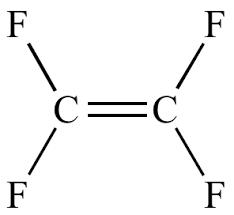QUESTION: 4

Arrange the following compounds in the order of leaving group ability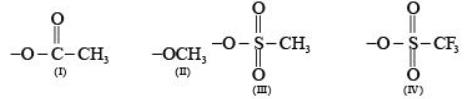Solution:

Weaker the base, better is the leaving group. Hence, basicities decrease in the order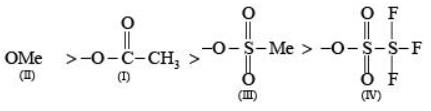Therefore, the order of leaving group ability decreases in the reverse order (IV) > (III) > (I) > (II).

QUESTION: 5

Most basic out of the following is

Solution:

No resonance and lone pair of electrons on sp3 hybridized N atom.

QUESTION: 6

Identify ‘Y’ in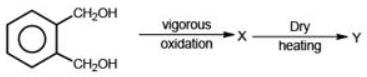Solution: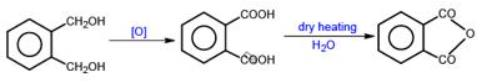QUESTION: 7

Identify the products A and B of the following reaction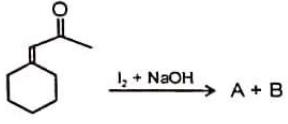Solution:

Iodoform reaction: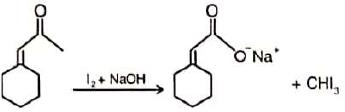QUESTION: 8

The strongest acid among the following aromatic compounds is

Solution:

Nitro is strongly electron withdrawing group. At ortho position intramolecular hydrogen bond reduces the acidic strength.

QUESTION: 9

In which of the following, gauche form is more stable than anti form of conformers?

Solution:

Gauche form can be more stable when intramolecular H-bonding occurs.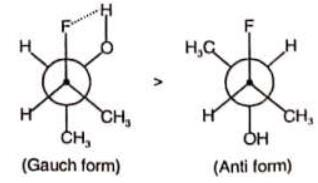QUESTION: 10

Which of the following derivatives of benzene would undergo hydrolysis most readily with aq. KOH?

Solution:

– NO2  group increases the reactivity in nucleophilic substitution on benzene ring due to –R and –I effect.

QUESTION: 11

The compound showing maximum number of geometrical isomers is

Solution:

More number of ligands.

QUESTION: 12

EAN rule is followed by

Solution:

EAN = 26- 2 + 6 x 2 = 36

QUESTION: 13

Aqueous CuSO4 solution is blue in colour. Which colour is absorbed by it ?

Solution:

Complementary colour.

The aqueous solution of CuSO4 consist of the complex [Cu(H2O)4]2+ ion which absorbed in orange-red region and impart deep blue colouration to solution.

QUESTION: 14

Which of the following elements can form square planar fluoride?

Solution:
• XeF4 is square planner.
• The shape of XeF4  is square planar. The central Xe atom has 4 bond pairs of electrons and two lone pairs of electrons.
• It undergoes sp3d2 hybridization which results in octahedral electron geometry and square planar molecular geometry.
• The two lone pairs are at opposite corners of an octahedron. This minimizes repulsion between them.
QUESTION: 15

Transition temperature of rhombic sulphur to monoclinic sulphur is

Solution: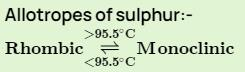QUESTION: 16

Which will have P-P bond?

Solution: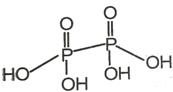QUESTION: 17

Least amount of carbon is present in

Solution:

Wrought iron is an iron alloy with a very low carbon content (less than 0.08%) in contrast to that of cast iron (2.1% to 4%).

QUESTION: 18

During electrophoresis of purple of cassius, the colloidal particles will

Solution:

Gold sol is negatively charged.

QUESTION: 19

In a reaction, 64.2 M of reactant becomes 32.1 M in 20 second. After 30 second from the start of the reaction 16.05 M is left. The value of rate constant will be

Solution:

It is zero order reaction,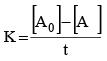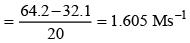QUESTION: 20

Correct comparison of ΔTb of the following (aqueous solution) is
1. 0.1 N NaCl
2. 0.1 N CaCl2
3. 0.1 N AlCl3
4. 0.1 N AI2 (SO)4

Solution:

As no of ions increases, lowering of VP increases.
here:

• NaCl = 2 ion
• CaCl2​  = 3 ion
• AlCl  = 4 ions
• Al2​(SO4​)3​ = 5 ions

So, correct order is (1) > (2) > (3) > (4)